# Civil Engineering - Applied Mechanics

### Exercise :: Applied Mechanics - Section 2

16.

The ratio of the ranges on the inclined plane with motion upward and with motion downward for a given velocity, angle of projection will be

 A.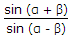B.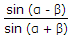C.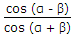D.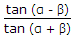Explanation:

No answer description available for this question. Let us discuss.

17.

For maximum range of a projectile, the angle of projection should be

 A. 30° B. 45° C. 60° D. none of these.

Explanation:

No answer description available for this question. Let us discuss.

18.

M.I. of a thin ring (external diameter D, internal diameter d) about an axis perpendicular to the plane of the ring, is

 A.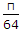(D4 + d4) B.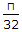(D4 - d4) C.(D4 + d4) D.(D4 x d4).

Explanation:

No answer description available for this question. Let us discuss.

19.

Pick up the correct statement from the following for the structure shown in below figure.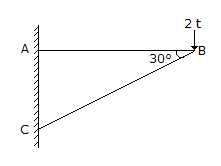A. The horizontal reaction at A is 23t ← B. The horizontal reaction at C is 23t → C. The vertical reaction at A is zero D. The vertical reaction at C is 2 t ↑ E. All the above.

Explanation:

No answer description available for this question. Let us discuss.

20.

A particle moves with a velocity of 2 m/sec in a straight line with a negative acceleration of 0.1 m/sec2. Time required to traverse a distance of 1.5 m, is

 A. 40 sec B. 30 sec C. 20 sec D. 15 sec E. 10 sec.